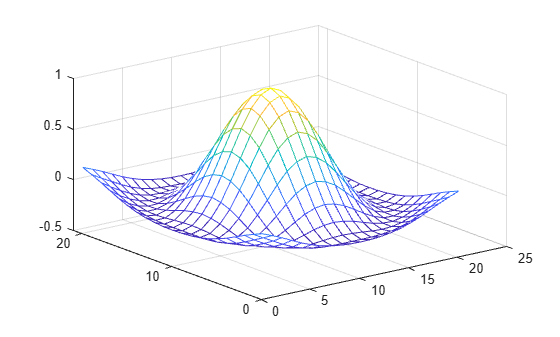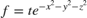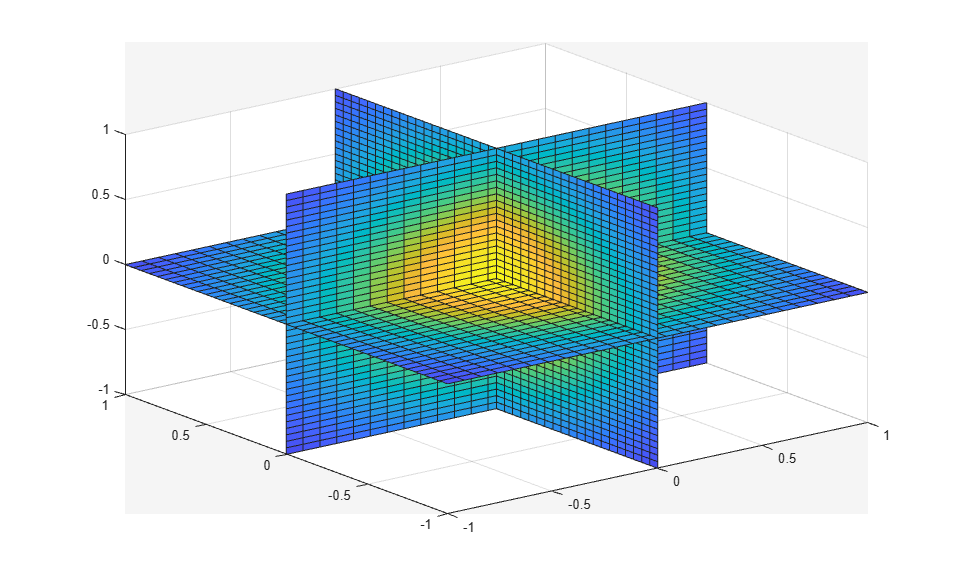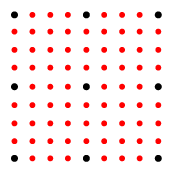# interpn

Interpolation for 1-D, 2-D, 3-D, and N-D gridded data in ndgrid format

## Syntax

``Vq = interpn(X1,X2,...,Xn,V,Xq1,Xq2,...,Xqn)``
``Vq = interpn(V,Xq1,Xq2,...,Xqn)``
``Vq = interpn(V)``
``Vq = interpn(V,k)``
``Vq = interpn(___,method)``
``Vq = interpn(___,method,extrapval)``

## Description

example

````Vq = interpn(X1,X2,...,Xn,V,Xq1,Xq2,...,Xqn)` returns interpolated values of a function of n variables at specific query points using linear interpolation. The results always pass through the original sampling of the function. `X1,X2,...,Xn` contain the coordinates of the sample points. `V` contains the corresponding function values at each sample point. `Xq1,Xq2,...,Xqn` contain the coordinates of the query points.```
````Vq = interpn(V,Xq1,Xq2,...,Xqn)` assumes a default grid of sample points. The default grid consists of the points, 1,2,3,...ni in each dimension. The value of ni is the length of the ith dimension in `V`. Use this syntax when you want to conserve memory and are not concerned about the absolute distances between points.```
````Vq = interpn(V)` returns the interpolated values on a refined grid formed by dividing the interval between sample values once in each dimension.```

example

````Vq = interpn(V,k)` returns the interpolated values on a refined grid formed by repeatedly halving the intervals `k` times in each dimension. This results in `2^k-1` interpolated points between sample values.```

example

````Vq = interpn(___,method)` specifies an alternative interpolation method: `'linear'`, `'nearest'`, `'pchip'`,`'cubic'`, `'makima'`, or `'spline'`. The default method is `'linear'`.```

example

````Vq = interpn(___,method,extrapval)` also specifies `extrapval`, a scalar value that is assigned to all queries that lie outside the domain of the sample points. If you omit the `extrapval` argument for queries outside the domain of the sample points, then based on the `method` argument `interpn` returns one of the following: The extrapolated values for the `'spline'` and `'makima'` methods`NaN` values for other interpolation methods ```

## Examples

collapse all

Define the sample points and values.

```x = [1 2 3 4 5]; v = [12 16 31 10 6];```

Define the query points, `xq`, and interpolate.

```xq = (1:0.1:5); vq = interpn(x,v,xq,'cubic');```

Plot the result.

```figure plot(x,v,'o',xq,vq,'-'); legend('Samples','Cubic Interpolation');```Create a set of grid points and corresponding sample values.

```[X1,X2] = ndgrid((-5:1:5)); R = sqrt(X1.^2 + X2.^2)+ eps; V = sin(R)./(R);```

Interpolate over a finer grid using `ntimes=1`.

```Vq = interpn(V,'cubic'); mesh(Vq);```Create the grid vectors, `x1`, `x2`, and `x3`. These vectors define the points associated with the values in `V`.

```x1 = 1:100; x2 = (1:50)'; x3 = 1:30;```

Define the sample values to be a 100-by-50-by-30 random number array, `V`. Use the `gallery` function to create the array.

```rng('default') V = rand(100,50,30);```

Evaluate `V` at three points outside the domain of `x1`, `x2`, and `x3`. Specify `extrapval = -1`.

```xq1 = [0 0 0]; xq2 = [0 0 51]; xq3 = [0 101 102]; vq = interpn(x1,x2,x3,V,xq1,xq2,xq3,'linear',-1)```
```vq = 1×3 -1 -1 -1 ```

All three points evaluate to `-1` because they are outside the domain of `x1`, `x2`, and `x3`.

Define an anonymous function that represents.

```f = @(x,y,z,t) t.*exp(-x.^2 - y.^2 - z.^2); ```

Create a grid of points in. Then, pass the points through the function to create the sample values, `V`.

```[x,y,z,t] = ndgrid(-1:0.2:1,-1:0.2:1,-1:0.2:1,0:2:10); V = f(x,y,z,t); ```

Now, create the query grid.

```[xq,yq,zq,tq] = ... ndgrid(-1:0.05:1,-1:0.08:1,-1:0.05:1,0:0.5:10); ```

Interpolate `V` at the query points.

```Vq = interpn(x,y,z,t,V,xq,yq,zq,tq); ```

Create a movie to show the results.

```figure('renderer','zbuffer'); nframes = size(tq, 4); for j = 1:nframes slice(yq(:,:,:,j),xq(:,:,:,j),zq(:,:,:,j),... Vq(:,:,:,j),0,0,0); caxis([0 10]); M(j) = getframe; end movie(M); ```## Input Arguments

collapse all

Sample grid points, specified as real arrays or vectors. The sample grid points must be unique.

• If `X1,X2,...,Xn` are arrays, then they contain the coordinates of a full grid (in ndgrid format). Use the `ndgrid` function to create the `X1,X2,...,Xn` arrays together. These arrays must be the same size.

• If `X1,X2,...,Xn` are vectors, then they are treated as grid vectors. The values in these vectors must be strictly monotonic, either increasing or decreasing.

Example: `[X1,X2,X3,X4] = ndgrid(1:30,-10:10,1:5,10:13)`

Data Types: `single` | `double`

Sample values, specified as a real or complex array. The size requirements for `V` depend on the size of `X1,X2,...,Xn`:

• If `X1,X2,...,Xn` are arrays representing a full grid (in `ndgrid` format), then the size of `V` matches the size of any array, `X1,X2,...,Xn`.

• If `X1,X2,...,Xn` are grid vectors, then `V` is an array whose `i`th dimension is the same length as grid vector `Xi`, where `i= 1,2,...n`.

If `V` contains complex numbers, then `interpn` interpolates the real and imaginary parts separately.

Example: `rand(10,5,3,2)`

Data Types: `single` | `double`
Complex Number Support: Yes

Query points, specified as a real scalars, vectors, or arrays.

• If `Xq1,Xq2,...,Xqn` are scalars, then they are the coordinates of a single query point in Rn.

• If `Xq1,Xq2,...,Xqn` are vectors of different orientations, then `Xq1,Xq2,...,Xqn` are treated as grid vectors in Rn.

• If `Xq1,Xq2,...,Xqn` are vectors of the same size and orientation, then `Xq1,Xq2,...,Xqn` are treated as scattered points in Rn.

• If `Xq1,Xq2,...,Xqn` are arrays of the same size, then they represent either a full grid of query points (in `ndgrid` format) or scattered points in Rn.

Example: `[X1,X2,X3,X4] = ndgrid(1:10,1:5,7:9,10:11)`

Data Types: `single` | `double`

Refinement factor, specified as a real, nonnegative, integer scalar. This value specifies the number of times to repeatedly divide the intervals of the refined grid in each dimension. This results in `2^k-1` interpolated points between sample values.

If `k` is `0`, then `Vq` is the same as `V`.

`interpn(V,1)` is the same as `interpn(V)`.

The following illustration depicts `k=2` in R2. There are 72 interpolated values in red and 9 sample values in black.Example: `interpn(V,2)`

Data Types: `single` | `double`

Interpolation method, specified as one of the options in this table.

`'linear'`The interpolated value at a query point is based on linear interpolation of the values at neighboring grid points in each respective dimension. This is the default interpolation method.C0
• Requires at least two grid points in each dimension

• Requires more memory than `'nearest'`

`'nearest'`The interpolated value at a query point is the value at the nearest sample grid point. Discontinuous
• Requires two grid points in each dimension.

• Fastest computation with modest memory requirements

`'pchip'`Shape-preserving piecewise cubic interpolation (for 1-D only). The interpolated value at a query point is based on a shape-preserving piecewise cubic interpolation of the values at neighboring grid points.C1
• Requires at least four points

• Requires more memory and computation time than `'linear'`

`'cubic'`The interpolated value at a query point is based on a cubic interpolation of the values at neighboring grid points in each respective dimension. The interpolation is based on a cubic convolution.C1
• Grid must have uniform spacing in each dimension, but the spacing does not have to be the same for all dimensions

• Requires at least four points in each dimension

• Requires more memory and computation time than `'linear'`

`'makima'`Modified Akima cubic Hermite interpolation. The interpolated value at a query point is based on a piecewise function of polynomials with degree at most three evaluated using the values of neighboring grid points in each respective dimension. The Akima formula is modified to avoid overshoots.C1
• Requires at least 2 points in each dimension

• Produces fewer undulations than `'spline'`

• Computation time is typically less than `'spline'`, but the memory requirements are similar

`'spline'`The interpolated value at a query point is based on a cubic interpolation of the values at neighboring grid points in each respective dimension. The interpolation is based on a cubic spline using not-a-knot end conditions.C2
• Requires four points in each dimension

• Requires more memory and computation time than `'cubic'`

Function value outside domain of `X1,X2,...,Xn`, specified as a real or complex scalar. `interpn` returns this constant value for all points outside the domain of `X1,X2,...,Xn`.

Example: `5`

Example: `5+1i`

Data Types: `single` | `double`
Complex Number Support: Yes

## Output Arguments

collapse all

Interpolated values, returned as a real or complex scalar, vector, or array. The size and shape of `Vq` depends on the syntax you use and, in some cases, the size and value of the input arguments.

SyntaxesSpecial ConditionsSize of VqExample
`interpn(X1,...,Xn,V,Xq1,...,Xqn)`
`interpn(V,Xq1,...,Xqn)`
and variations of these syntaxes that include `method` or `extrapval`
`Xq1,...,Xqn` are scalarsScalar`size(Vq) = [1 1]` when you pass `Xq1,...,Xqn` as scalars.
Same as above`Xq1,...,Xqn` are vectors of the same size and orientationVector of same size and orientation as `Xq1,...,Xqn`In 3-D, if
`size(Xq1) = [100 1]`,
and `size(Xq2) = [100 1]`,
and ```size(Xq3) = [100 1]```,
then ```size(Vq) = [100 1]```.
Same as above`Xq1,...,Xqn` are vectors of mixed orientation`size(Vq) = [length(Xq1),...,length(Xqn)]`In 3-D, if
`size(Xq1) = [1 100]`,
and `size(Xq2) = [50 1]`,
and ```size(Xq3) = [1 5]```,
then ```size(Vq) = [100 50 5]```.
Same as above`Xq1,...,Xqn` are arrays of the same sizeArray of the same size as `Xq1,...,Xqn`In 3-D, if
`size(Xq1) = [50 25]`,
and `size(Xq2) = [50 25]`,
and ```size(Xq3) = [50 25]```,
then ```size(Vq) = [50 25]```.
`interpn(V,k)`
and variations of this syntax that include `method` or `extrapval`
None

Array in which the length of the `i`th dimension is
`2^k * (size(V,i)-1)+1`,

In 3-D, if
`size(V) = [10 12 5]`,
and `k = 3`,
then ```size(Vq) = [73 89 33]```.

collapse all

### Strictly Monotonic

A set of values that are always increasing or decreasing, without reversals. For example, the sequence, ```a = [2 4 6 8]``` is strictly monotonic and increasing. The sequence, ```b = [2 4 4 6 8]``` is not strictly monotonic because there is no change in value between `b(2)` and `b(3)`. The sequence, `c = [2 4 6 8 6]` contains a reversal between `c(4)` and `c(5)`, so it is not monotonic at all.

### Full Grid (in ndgrid Format)

For `interpn`, the full grid consists of n arrays, `X1,X2,...,Xn`, whose elements represent a grid of points in Rn. The ith array, `Xi`, contains strictly monotonic, increasing values that vary most rapidly along the ith dimension.

Use the `ndgrid` function to create a full grid that you can pass to `interpn`. For example, the following code creates a full grid in R2 for the region, 1 ≤ X1 ≤ 3, 1≤ X2 ≤ 4.

`[X1,X2] = ndgrid(-1:3,(1:4))`
```X1 = -1 -1 -1 -1 0 0 0 0 1 1 1 1 2 2 2 2 3 3 3 3 X2 = 1 2 3 4 1 2 3 4 1 2 3 4 1 2 3 4 1 2 3 4```

### Grid Vectors

For `interpn`, grid vectors consist of n vectors of mixed-orientation that define the points of a grid in Rn.

For example, the following code creates the grid vectors in R3 for the region, 1 ≤ x1 ≤ 3, 4 ≤ x2 ≤ 5, and 6 ≤x3≤ 8:

```x1 = 1:3; x2 = (4:5)'; x3 = 6:8;```

### Scattered Points

For `interpn`, scattered points consist of n arrays or vectors, `Xq1,Xq2,...,Xqn`, that define a collection of points scattered in Rn. The `i`th array, `Xi`, contains the coordinates in the `i`th dimension.

For example, the following code specifies the points, (1, 19, 10), (6, 40, 1), (15, 33, 22), and (0, 61, 13) in R3.

```Xq1 = [1 6; 15 0]; Xq2 = [19 40; 33 61]; Xq3 = [10 1; 22 13];```

## Version History

Introduced before R2006a